﻿Kaufman

# Kaufman

The Kaufman's Adaptive Moving Average (Kama) indicator was developed to address the problem of market noise when identifying trends.  The indicator, in its essence, is an Exponential Moving Average (Ema) that automatically becomes more sensible (fast) or robust (slow) depending upon the conditions that prevail in the market.  On a trending market, there are relatively small swings, so Kama shorts the Ema period and follows the price very closely.  If the market moves sideways then Kama increases the Ema period to eliminate market noise and follows the price slowly.

To measure the strength of a trend, Kaufman introduced the Efficiency Ratio (ER) that compares price direction with the level of volatility.  The ER value defined within the range [0 … 1] is then used to regulate the Ema smoothing period from the minimal (fast) to the maximal (slow) value.Market Signals

Kama informs about trends prevailing on the market:

• Rising or falling Kama indicates an upwards or downwards trend, respectively.

• In a range-bound market, Kama runs almost horizontally.Calculation
1. Calculate the Efficiency Ratio over the period N specified: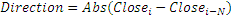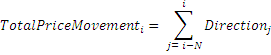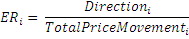2. Calculate the Smoothing Constant (SC):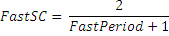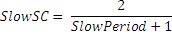3. Calculate Kama value: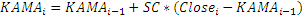Chart Example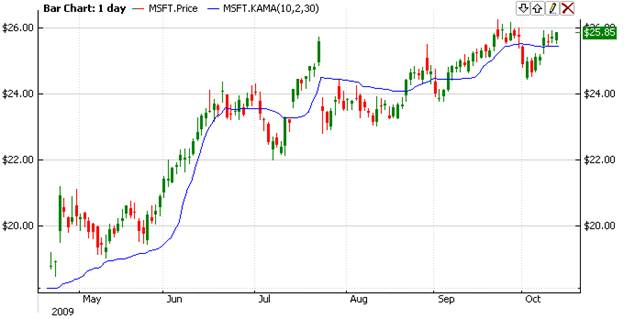Implementation and Usage

To initialize Kama use one of the following constructors:

Kama – sets default values: period = 10, fastPeriod = 2, slowPeriod = 30

Kama(Int32)- sets value for period, others are default

Kama(Int32, Int32, Int32) - sets values for periods

Use

KAMA - property to get current value.Example
C#
``` 1// Create new instance
2Kama kama = new Kama(28, 6, 30);
3
4// Number of stored values
5kama.HistoryCapacity = 2;
6